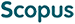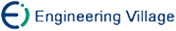李胜梅, 程步奇, 高兴誉, 乔林, 汤志忠. 基于非线性回归方程偏导数分析应用程序性能敏感度的方法[J]. 计算机研究与发展, 2010, 47(9): 1654-1662.
 引用本文: 李胜梅, 程步奇, 高兴誉, 乔林, 汤志忠. 基于非线性回归方程偏导数分析应用程序性能敏感度的方法[J]. 计算机研究与发展, 2010, 47(9): 1654-1662.Li Shengmei, Cheng Buqi, Gao Xingyu, Qiao Lin, Tang Zhizhong. A Method on Analyzing Performance Sensitivity of Applications Based on Partial Derivatives of Non-linear Regression Equation[J]. Journal of Computer Research and Development, 2010, 47(9): 1654-1662.
 Citation: Li Shengmei, Cheng Buqi, Gao Xingyu, Qiao Lin, Tang Zhizhong. A Method on Analyzing Performance Sensitivity of Applications Based on Partial Derivatives of Non-linear Regression Equation[J]. Journal of Computer Research and Development, 2010, 47(9): 1654-1662.## A Method on Analyzing Performance Sensitivity of Applications Based on Partial Derivatives of Non-linear Regression Equation

• 摘要: 性能敏感度反映了应用程序性能相对于性能影响因素的变化率，对性能敏感度的量化分析可为体系结构设计和程序性能优化提供有意义的参考和指导.提出了一种分析程序性能敏感度的非线性回归模型(PS-NLRM)，能够量化不同应用程序的性能敏感度.通过主成分分析消除了影响性能的性能事件之间的相关性，通过曲线拟合引入非线性项，建立了程序性能CPI和性能事件之间的非线性回归方程.模型应用在SPEC CPU2006整型程序之上，通过了t检验和F检验，达到90%以上的拟合度.基于非线性回归方程相对于性能事件的偏导数，得到不同应用程序的性能对性能事件的敏感度.利用性能敏感度对SPEC CPU2006整型程序性能进行预测的平均相对误差约为4.5%，比传统线性回归模型预测误差下降50%.

Abstract: Performance sensitivity reflects how sensitive the performance is to the influence factors. Analysis on performance sensitivity of different applications can guide the architects on the architecture design and help programmers on application optimization. In this paper, a performance sensitivity non-linear regression model (PS-NLRM) is set up to quantitatively analyze the performance sensitivity of different applications. In the model, principal components analysis is used to eliminate the linear correlations among influence factors which are quantified with performance events. Non-linear independent variables are introduced by curve fitting in the model. By regression analysis, a non-linear regression model is set up between cycles per instruction (CPI) and performance events. The model is implemented in SPEC CPU2006 integer benchmarks and uses the benchmarks as samples. The model is verified by t test and F test with goodness of fit over 90%. By using the partial derivatives of the non-linear regression equation of the model, performance sensitivity is obtained which is denoted by the quantitative change of CPI with the corresponding changes of the performance events. Based on performance sensitivity, performance of applications can be predicted. The average relative error of predicted performance of SPEC CPU2006 integer benchmarks is about 4.5%, which is half reduced compared with the traditional linear regression models./下载:  全尺寸图片 幻灯片
• 分享
• 用微信扫码二维码

分享至好友和朋友圈